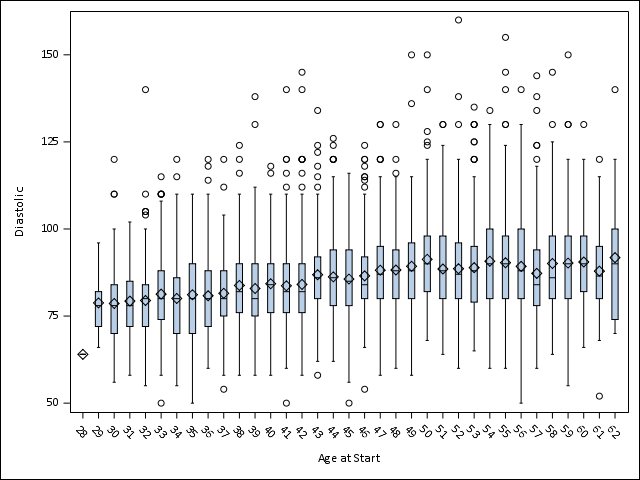## How do i make the tick mark value smaller?

Hi guys,

I am using gplot to graph my experience data, with x-axis ranger from -1 to 22 by step of 1. Everything work out nicely, however one problem is that I only have limited space across a-axis. for example for double digits number 10, 11, 12... the number is displayed vertically like:

1 1 1

0 1 2

So I am asking how do I make the font smaller that I can fit in all the values horizontally.

Thanks.

1 ACCEPTED SOLUTION

Accepted Solutions

## Re: How do i make the tick mark value smaller?

Here's how to control the font height of the tickmark values along an axis, in gplot:

axis1 value=(height=1.0pct);

proc gplot data=sashelp.class;
plot height*weight / haxis=axis1;
run;

3 REPLIES 3

## Re: How do i make the tick mark value smaller?

You should use SGPLOT instead of GPLOT, then add an XAXIS statement and you can specify the values a little easier. I would recommend skipping every other one instead of changing the font.

``xaxis values = (-2 to 22 by 2);``

GPLOT is part of SAS/GRAPH and fairly old, the graphics are not as nice and it's harder to work with, which is why I recommend SGPLOT instead.

@ljr4616 wrote:

Hi guys,

I am using gplot to graph my experience data, with x-axis ranger from -1 to 22 by step of 1. Everything work out nicely, however one problem is that I only have limited space across a-axis. for example for double digits number 10, 11, 12... the number is displayed vertically like:

1 1 1

0 1 2

So I am asking how do I make the font smaller that I can fit in all the values horizontally.

Thanks.PGStats
Opal | Level 21

## Re: How do i make the tick mark value smaller?

Look what SGPLOT does in this simple example (version 9.4 TS Level 1M5) :

``````proc sgplot data=sashelp.heart;
vbox diastolic / category=ageAtStart;
run;``````PG

## Re: How do i make the tick mark value smaller?

Here's how to control the font height of the tickmark values along an axis, in gplot:

axis1 value=(height=1.0pct);

proc gplot data=sashelp.class;
plot height*weight / haxis=axis1;
run;

Discussion stats
• 3 replies
• 713 views
• 1 like
• 4 in conversation